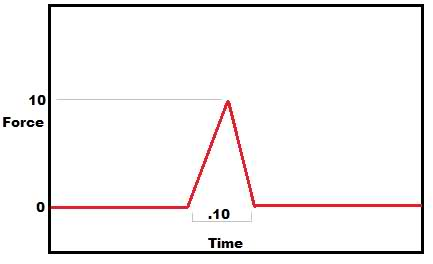# Impulse Momentum (et al.) test

## Homework Statement

I took a test today and I am pretty sure I got everything right except for this one problem that was on there which I am unsure of. If I remember correctly, it went like this:

It said there was a particle of mass 2 kg moving with a speed of 5 m/s to the right. Then there was a graph that more or less looked like this one I just made:The question asked what the final velocity was after the impulse.

## Homework Equations

I believe this is correct and relevant.
$$\int F dt = J = ΔP = mv_{f} - mv_{i}$$

## The Attempt at a Solution

I wagered that since the impulse was equal to the area under the curve on a Force v. Time graph, that the problem could be done in this way:

$$J = \frac{1}{2}(base)(height)$$
$$J = \frac{1}{2}(0.10)(10)$$
$$J = 0.5$$

$$J = mv_{f} - mv_{i}$$
$$0.5 = (2)v_{f} - (2)(5)$$
$$10.5 = (2)v_{f}$$
$$5.25 = v_{f}$$

So I said the velocity afterwards was 5.25 m/s (and part b asked the direction, which I claimed was to the right).

It's unlike any practice/hw/quiz problems we have had before and there was some equation she wrote on the board:
$$F_{avg}Δt = mv_{f} - mv_{i}$$

Which I believe is basically what I did, I just didn't use that equation.

Did I mess up?

Doc Al
Mentor
Assuming that the impulse was also to the right, you did fine.

FYI: You could have used your instructor's formula; In this case the average force is 10/2 = 5 N. Same answer, of course. (Since it's really the same thing.)

Whew, thank you.

Another question that I am a little sketchy about is one where they showed some function U(x) which represented potential energy with respect to distance.The mass of the thing was m kg The question(s) were:

a.)What is force:
I simply took the derivative of U, and my final answer was U'(x). Sorry I can't remember the function, but it was something that had the general shape of y = 1/x.

b.)If the object was released from it's origin, what is it's speed at x = 2?

I claimed that mgU(0) was the total energy on the system, and so, after the thing fell or whatever, the final velocity could be found via:

$$mgU(0) = mgU(2) + \frac{1}{2}mv^{2}$$
Solving for v obviously.

?

Doc Al
Mentor
a.)What is force:
I simply took the derivative of U, and my final answer was U'(x). Sorry I can't remember the function, but it was something that had the general shape of y = 1/x.
Almost. Given a potential energy function, the associated force is given by -dU/dx. The minus sign is important.

b.)If the object was released from it's origin, what is it's speed at x = 2?

I claimed that mgU(0) was the total energy on the system, and so, after the thing fell or whatever, the final velocity could be found via:

$$mgU(0) = mgU(2) + \frac{1}{2}mv^{2}$$
Solving for v obviously.
U(x) is the potential energy, not mgU(x). (You're confusing this with a gravitational PE problem, perhaps?)

You want:
$$U(0) = U(2) + \frac{1}{2}mv^{2}$$

Oh ok, I got confused trying to replicate the problem from memory into latex.

Yes I used $F = -\frac{dU}{dx}$
and I did use U(x) for potential, not mgU(x).

Doc Al
Mentor
Oh ok, I got confused trying to replicate the problem from memory into latex.

Yes I used $F = -\frac{dU}{dx}$
and I did use U(x) for potential, not mgU(x).
Good!